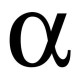# Answer to Approximation Question with Explanation:

by on December 7th, 2009

The answer to yesterday’s question.  Try the problem first if you haven’t done so already and click more to see if you got the answer right.

The ratio of 2 + √6  to 3 is approximately equal to which of the following ratios?

A. 2:1

B. 3:2

C. 4:3

D. 7:6

E. 13:12

This looks like a very difficult question, but there’s an underlying logic to it all if you stop to consider what information is provided in both the stimulus and in the answer choices.

The only calculating we need to do is √6, which we know is greater than 2 but less than 3. We can begin to approximate by using the midpoint between 2 and 3, and squaring it to see how close to 6 we get.  2.52 (the same thing as 252, just move the decimal) = 6.25, which is slightly too high. An attempt with 2.42 yields 5.76. We can reasonably draw the conclusion that √6 is between 2.4 and 2.5. We can stop at 2.45, which should be close enough.

Entering √6 = 2.45 into the ratio, we approximate it to be 2 + 2.45 to 3, or.

Before we move on, let’s find a few handy equivalents to that ratio:is equivalent to,, and, and multiplies by a factor of 10 to equal. Only go so far as you need in order to convince yourself that 3:2 is the best option.

Instead of doing mindless calculations of fractions-to-decimals all day and night, consider the relationships among the answer choices in terms of inequalities. In choices A through C, we have:### If you liked this article, let Jake Becker know by clicking Like.

•I did it this way:

(2+√6)/3 = 2/3 + √6/3 = 0.66 + √6/3

Now √6/3 = (√3√2)/(√3√3) = √(2/3) ≈ √(.66) ≈ 0.8

So the original ratio is approximately 0.66 + 0.80 = 1.46

(A) is clearly far off. (B) is very close. I still checked (C). Recognizing the pattern in the answer choices helped me eliminate.

•that is exactly what i did in abt a minute. sqr.rt 2 and 3 are easier to deal with since I already know their value. However, instead of doing sq.rt. of 0.66, i went with 1.414/1.732 which approx. to 14/18 and is abt 0.80. you add it with 2/3 and get 1.46. Also, since we increase the denominator here, we can guess that the approx. value would be on the higher side which gives us 3/2

•I made a rather rougher approximation and still ended up with the right answer.

I visualized the following:
4 5 6 7 8 9
2 x 3

And then noted that 6 was approximately 2/5 from 4 to 9.

2 + (2/5) = 2.4 + 2 = 4.4/3 => closest to 3/2, B.